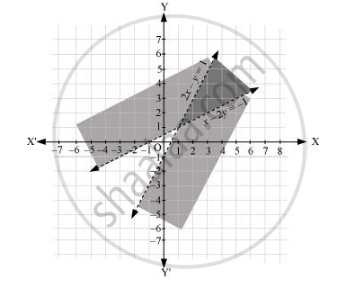# Solve the Following System of Inequalities Graphically: 2x – Y > 1, X – 2y < –1 - Mathematics

Solve the following system of inequalities graphically: 2x – y > 1, x – 2y < –1

#### Solution

2x – y > 1 … (1)

x – 2y < –1 … (2)

The graph of the lines, 2x – y = 1 and x – 2= –1, are drawn in the figure below.

Inequality (1) represents the region below the line, 2x – y = 1 (excluding the line 2x – y = 1), and inequality (2) represents the region above the line, x – 2= –1 (excluding the line x – 2= –1).

Hence, the solution of the given system of linear inequalities is represented by the common shaded region excluding the points on the respective lines as follows.Concept: Solution of System of Linear Inequalities in Two Variables
Is there an error in this question or solution?
Chapter 6: Linear Inequalities - Exercise 6.3 [Page 129]

#### APPEARS IN

NCERT Class 11 Mathematics
Chapter 6 Linear Inequalities
Exercise 6.3 | Q 5 | Page 129

Share